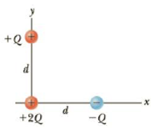Chapter 15, Problem 61AP

Chapter
Section
Textbook Problem

A point charge +2Q is at the origin and a point charge −Q is located along the x-axis at x = d as in Figure P15.61. Find symbolic expressions for the components of the net force on a third point charge +Q located along the y-axis at y = d.Figure P15.61

To determine
The components of electric force.

Explanation

The free body diagram is,

• F+ is the force due to +2Q charge.
• F is the force due to –Q charge.
• r is the distance between +2Q charge and –Q charge.

From the diagram,

r=2d

Formula to calculate F is,

F=ke(Q)(Q)r2=ke(Q)(Q)(2d)2=keQ22d2

Formula to calculate F+ is,

F+=ke(Q)(2Q)d2=2keQ2d2

From the diagram, the x component of electric force is,

Still sussing out bartleby?

Check out a sample textbook solution.

See a sample solution

The Solution to Your Study Problems

Bartleby provides explanations to thousands of textbook problems written by our experts, many with advanced degrees!

Get Started

Find more solutions based on key concepts